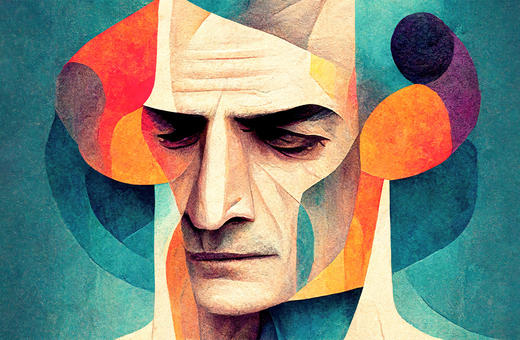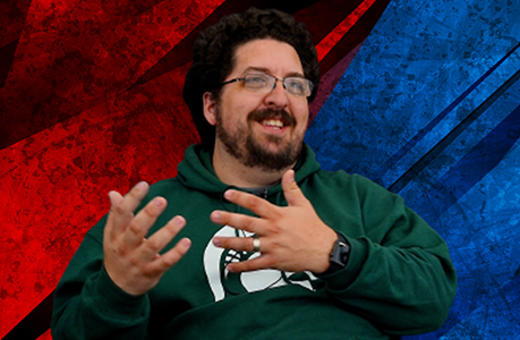# Most truths cannot be expressed in language

## Gödel and the infinity of unprovable truths

Gödel’s famous incompleteness theorem showed us that there is a statement in basic arithmetic that is true but can never be proven with basic arithmetic. But that is just the beginning of the story. There are more true but unprovable, or even able to be expressed, statements than we can possibly imagine, argues Noson S. Yanofsky.

Mathematics has long been viewed as the great bastion of certainty. This absolute certainty comes from the notion of proof. We have confidence that what is proven from true axioms will always be true. Scientists and philosophers are envious of the mathematician’s certainty. Some philosophers, like Spinoza and Wittgenstein, even tried to mimic the lofty proofs of the mathematician in expressing their philosophical ideas.

Part of this certainty came to a halt in 1931 when a twenty-five-year-old Austrian logician named Kurt Gödel proved a revolutionary theorem that shook the foundations of mathematics to its core. The theorem --- called “Gödel’s incompleteness theorem” --- says that there is a statement in basic arithmetic that is true but can never be proven with basic arithmetic. Young Gödel demonstrated that proofs are not as pervasive as previously thought (see Figure 1.) Although this theorem is over ninety years old, its consequences are still remarkable and not fully explored.The argument that Gödel used is similar to the liar’s paradox:

“This sentence is false.”

Let us analyze this:

•         If the statement itself is true, then it says that it is false, and hence it is false.

•         If the statement itself is false, then it is false that it is false and hence it must be true.

So which is it? True or false? The answer is that the statement above can neither be true nor false. Along the same lines, Gödel showed that exact mathematical proofs about basic arithmetic could be made into statements about basic arithmetic. He used this method to formulate a mathematical statement that says

“This statement is not provable.”

Let us analyze this:

•         If the statement is true, then it is true and unprovable.

•         If the statement is false, then it is provable and hence true (because basic arithmetic can only prove true statements). But the statement says it is not provable. So, the statement is true and false at the same time. This cannot be.

___

There are infinitely many true but unprovable statements

___

One can easily see that this strange mathematical statement, by definition, is true and cannot be proven. No amount of ingenuity will ever prove this statement within basic arithmetic. It is not that we do not have the ability to prove it now. Rather, it will never be proven with the tools of basic arithmetic. This statement is called a “Gödel statement.”

As a bonus, Gödel described another interesting statement in the language of basic arithmetic. He was able to formulate the statement in basic arithmetic that says:

“Basic arithmetic cannot prove a contradiction.”

It turns out that this statement is also true but unprovable. This means that basic arithmetic cannot prove that basic arithmetic is free of contradictions.

The question immediately arises as to whether there are other mathematical statements that are true but unprovable.

For about forty years, these two unnatural mathematical statements were from the select few statements that were known to be true but unprovable with basic arithmetic. Most mathematicians ignored these logical results because the statements were so atypical and hence not the usual fare of mathematicians. Then in 1977 Jeff Paris and Leo Harrington found an interesting, real-world mathematical true statement about combinatorics which they showed could not be proven with basic arithmetic.

Gregory Chaitin described an innovative way of finding true but unprovable statements. He started by examining the complexity of the axioms of a logical system. He showed that there are certain statements that are much more complex than the axioms of the system. Such statements are true but cannot be proven by the axioms of the logical system. The following motto is sometimes used to explain this:

“A fifty-pound logical system cannot prove a seventy-five-pound theorem.”

In particular, basic arithmetic is a logical system that has a level of complexity, and so there are certain types of statements that are true but too complex to be proven using basic arithmetic. The main point of our story is that within basic arithmetic, we can always find more complicated statements of a certain type. Hence, there are infinitely many true but unprovable statements.

Cristian Calude extended Chaitin’s findings. He demonstrated that provable statements are actually very rare within the space of all true statements. In a sense, he showed that in the space of all true statements, every provable true statement is surrounded by many unprovable true statements.

___

Some true mathematical facts are expressible while the vast, vast majority of mathematical facts are inexpressible

___

This line of reasoning about the preponderance of true but unprovable statements can be extended, however first we must learn more about infinity. There are different types of infinities. Mathematicians at the end of the 19th century realized that the infinity of the natural numbers {0, 1, 2, 3,….} is of a much smaller type than the infinity of the real numbers. The real numbers contain all the natural numbers, but they also contain all fractions and all the real numbers that can only be expressed with an infinite number of decimal places. These two infinities are different sizes. We call any infinite set that is the same size as the natural numbers “countably infinite.” In contrast, any infinite set that is larger than the natural numbers, such as the real numbers, is called “uncountably infinite.” The main point to keep in mind is that uncountable infinite sets are vastly, vastly larger than countable infinite sets. In fact, we say that a countably infinite set is “vanishingly small” compared to an uncountably infinite set.

Some examples of sets that are countably infinite are the natural numbers, the rational numbers, and finite strings of symbols. In fact, both natural and mathematical language is comprised of finite strings of symbols and their collection of words, sentences, or expressions are countably infinite.

Some examples of sets that are uncountably infinite are the real numbers, the complex numbers, and sets of infinite strings of symbols. Another important uncountably infinite set is the collection of subsets of the natural numbers. Here are some subsets of natural numbers.

•           All natural numbers: {0,1,2,3, … }.

•           Prime numbers: {2,3,5,7,11,…}.

•           Even numbers: {0,2,4,6, …}.

•           The empty set: { }.

•           The set {5, 29, 16, 34, 27}.

•           The set that does not contain {5, 29, 16, 34, 27}.

•           Cubic numbers: {0,1,8,27, 64, …}.

•           etc.

As you can see, there are a lot of them. The collection of all such subsets is uncountably infinite.

Now that we have these different notions of infinity in our toolbox, let us apply them to our concept of true but unprovable statements. All language is countably infinite. The set of statements in basic arithmetic, the subset of true statements, and the subset of provable statements are all countably infinite.

This brings to light an amazing limitation of the power of language. The collection of all subsets of natural numbers is uncountably infinite while the set of expressions describing subsets of natural numbers is countably infinite. This means that the vast, vast majority of subsets of natural numbers cannot be expressed by language. The above examples of subsets of natural numbers are expressed by language, but they are part of the few rather than the many. The majority of the subsets are inexpressible. They defy language.

What about mathematical facts about subsets of natural numbers? For every subset S, one can ask if a number is a member of S. For any subset S, one can ask if S has a certain property. For any two subsets, S and T, one can ask if S is a subset of T. Each answer to such question is a mathematical fact. These are just some of the many mathematical facts about subsets of natural numbers. Because the set of subsets of natural numbers is uncountably infinite, and there are many mathematical facts about each subset, the set all mathematical facts is uncountably infinite. In stark contrast, the collection of all properties that can be expressed or described by language is only countably infinite because there is only a countably infinite collection of expressions. All the other properties of natural numbers cannot even be expressed. There are not enough expressions for them. They are beyond language. Let us call facts that we can describe with mathematical expressions “expressible.” The rest of the facts are “inexpressible” (see Figure 2). Some true mathematical facts are expressible while the vast, vast majority of mathematical facts are inexpressible.

___

We have come a long way since Gödel. A true but unprovable statement is not some strange, rare phenomenon. In fact, the opposite is correct. A fact that is true and provable is a rare phenomenon

___We have come a long way since Gödel. A true but unprovable statement is not some strange, rare phenomenon. In fact, the opposite is correct. A fact that is true and provable is a rare phenomenon. The collection of mathematical facts is very large and what is expressible and true is a small part of it. Furthermore, what is provable is only a small part of those.

We live in a golden age of mathematics. Many different fields of mathematics are being developed, new methods are constantly being invented, and a myriad of theorems are proven every day. Despite all the accomplishments in mathematics, we nevertheless have to be humbled by the immensity of the unfathomable mathematical universe.

Latest Releases